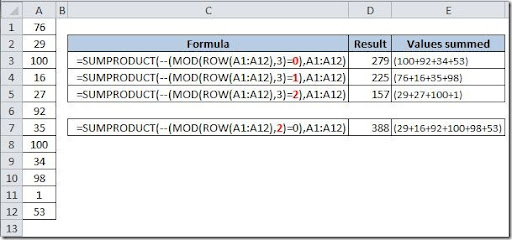### Sum every x Row

This can be very handy. Assume that you have your data on cells A1:A12 and you want to sum only, say, the values on each 3rd row. Using a SUMPRODUCT() formula like this:

=SUMPRODUCT(--(MOD(ROW(A1:A100),3)=0),A1:A100)

you can sum every 3rd row. You can adjust the 0 like this:

0 will sum every 3rd row
1 will sum every 3rd row starting with row 1
2 will sum every 3rd row starting with row 2

You can also adjust the 3 to change the number of rows to be summed. You can see here an example on the use of the multiple variations of this formula: